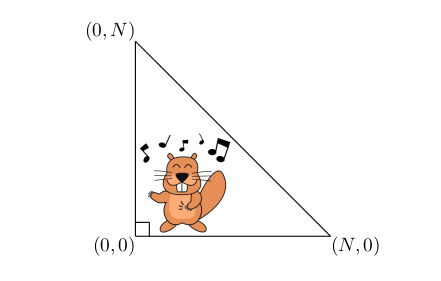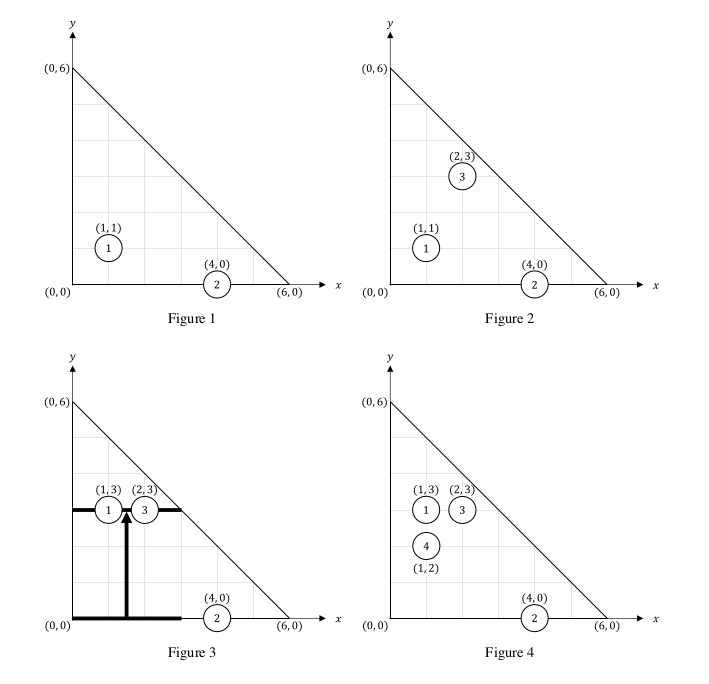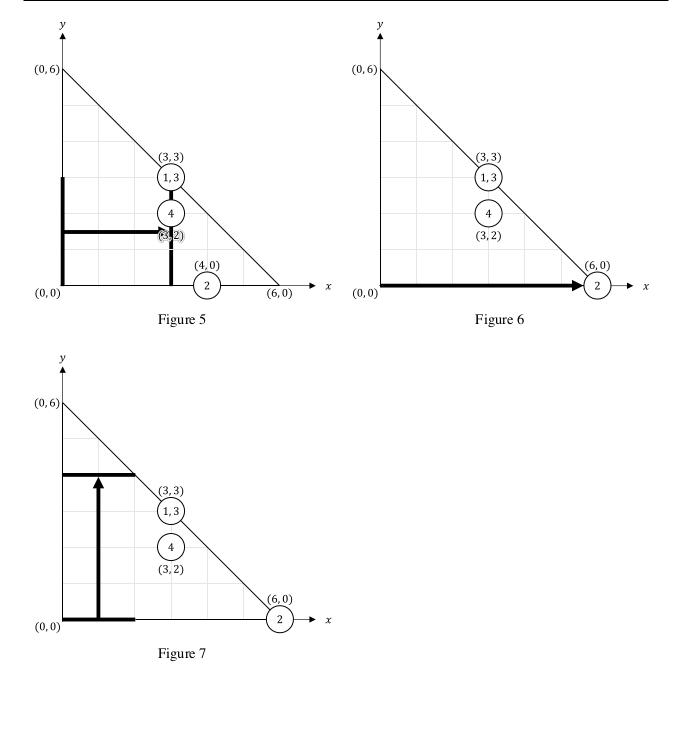# #503. 【JOISC2020】扫除• 比太郎把扫帚放在$y$轴上，使扫帚的一个端点落在原点。然后，他沿着$x$轴的方向水平移动扫帚，直到扫帚碰到房间的斜边为止，保持扫帚的一个端点在$x$轴上，并且与$y$轴平行。如果扫帚的宽度是$l$，原来位于$(x,y) (x < N - l,y \leq l)$的灰尘将会被扫到$(N-l,y)$处。（$(N-l,y)$处可能有其它灰尘。）这被称作过程H
• 比太郎把扫帚放在$x$轴上，使扫帚的一个端点落在原点。然后，他沿着$y$轴的方向竖直移动扫帚，直到扫帚碰到房间的斜边为止，保持扫帚的一个端点在$y$轴上，并且与$x$轴平行。如果扫帚的宽度是$l$，原来位于$(x,y) (x \leq l,y < N - l)$的灰尘将会被扫到$(x,N - l)$处。（$(x,N-l)$处可能有其它灰尘。）这被称作过程V

• 比太郎询问第$j$片灰尘的坐标
• 比太郎用宽度为$L_j$的扫帚进行过程H
• 比太郎用宽度为$L_j$的扫帚进行过程V
• 一片新的灰尘出现在了$(A_j,B_j)$处。如果原来有$c$片灰尘，新的灰尘的编号就是$c+1$

### 输入格式

• 如果$T_j=1$，这一行还含有一个整数$P_j$。这表示比太郎想查询第$j$片灰尘的坐标。
• 如果$T_j=2$，这一行还含有一个整数$L_j$。这表示比太郎用一个宽度为$L_j$的扫帚进行过程H。
• 如果$T_j=3$，这一行还含有一个整数$L_j$。这表示比太郎用一个宽度为$L_j$的扫帚进行过程V。
• 如果$T_j=4$，这一行还含有两个整数$A_j,B_j$。这表示$(A_j,B_j)$处出现了一片新的灰尘。

### 样例数据

#### input1

6 2 10
1 1
4 0
4 2 3
3 3
1 1
4 1 2
2 3
2 0
1 4
3 2
1 3
1 2

#### output1

1 3
3 2
3 3
6 0

#### 样例解释1

• 一开始，第一片灰尘落在$(1,1)$，第二片落在$(4,0)$，参照图$1$。
• 在第一个事件中，第三片灰尘出现在$(2,3)$，参照图$2$。
• 在第二个事件中，比太郎用宽度为$3$的扫帚进行过程V。第一片灰尘被移动到了$(1,3)$，参照图$3$。
• 在第三个事件中，比太郎计算第一片灰尘的坐标$(1,3)$。
• 在第四个事件中，第四片灰尘出现在了$(1,2)$处，参照图$4$。
• 在第五个事件中，比太郎用宽度为$3$的扫帚进行过程H。第一片灰尘的坐标现在是$(3,3)$，第三片灰尘的坐标现在是$(3,3)$，第四片灰尘的坐标现在是$(3,2)$，参照图$5$。
• 在第六个事件中，比太郎用宽度为$0$的扫帚进行过程H。第二片灰尘被移到了$(6,0)$，参照图$6$。
• 在第七个事件中，比太郎计算第$4$片灰尘的坐标$(3,2)$。
• 在第八个事件中，比太郎使用宽度为$2$的扫帚进行操作V。没有灰尘被移动，参照图$7$。
• 在第九个事件中，比太郎计算第三片灰尘的坐标$(3,3)$。
• 在第十个事件中，比太郎计算第二片灰尘的坐标$(6,0)$。#### input2

9 4 8
2 3
3 1
1 6
4 3
2 6
1 3
2 2
1 4
2 3
1 2
2 4
1 1

#### output2

3 6
4 3
7 1
6 3

#### input3

8 1 8
1 5
4 4 1
2 6
1 2
2 3
4 2 2
2 5
1 1
1 3

#### output3

4 1
3 5
3 2

### 限制与约定

• $1 \leq N \leq 1 000 000 000$
• $1 \leq M \leq 500 000$
• $1 \leq Q \leq 1 000 000$
• $0 \leq X_i \leq N (1 \leq i \leq M)$
• $0 \leq Y_i \leq N (1 \leq i \leq M)$
• $X_i + Y_i \leq N (1 \leq i \leq M)$
• 第$P_j$片灰尘存在
• $0 \leq L_j \leq N-1 (1 \leq j \leq Q)$
• $0 \leq A_j \leq N (1 \leq j \leq Q)$
• $0 \leq B_j \leq N (1 \leq j \leq Q)$
• $A_j + B_j \leq N (1 \leq j \leq Q)$
• 至少有一个$T_j=1$的操作。

### 子任务

1. ($1$ 分) $M \leq 2000, Q \leq 5000$
2. ($10$ 分) $T_j = 1,2,4$
3. ($11$ 分) $T_j = 1,2,3, X_j \leq X_{j+1}, Y_j \geq Y_{j+1} (1 \leq j \leq M-1)$
4. ($53$ 分) $T_j = 1,2,3$
5. ($25$ 分) 没有额外的限制